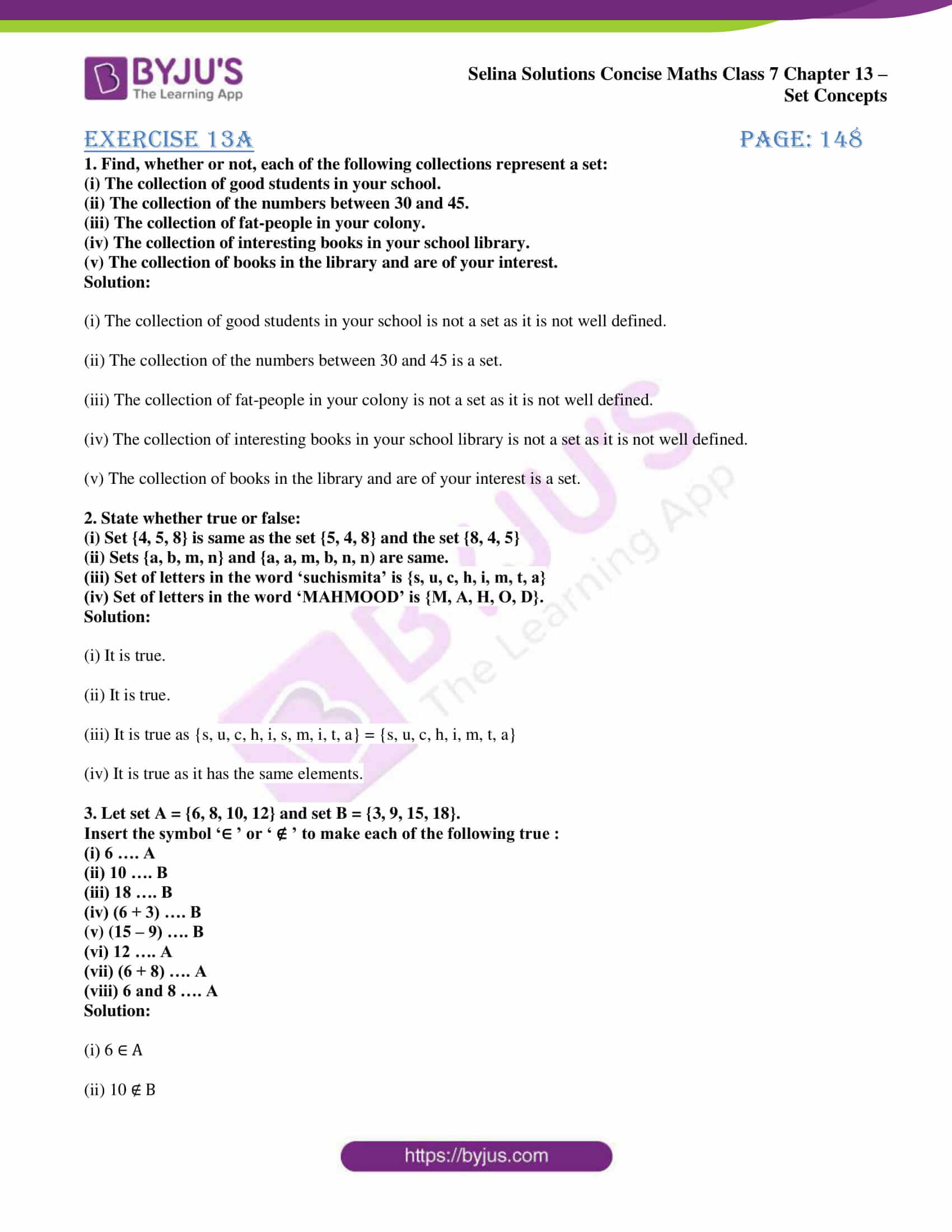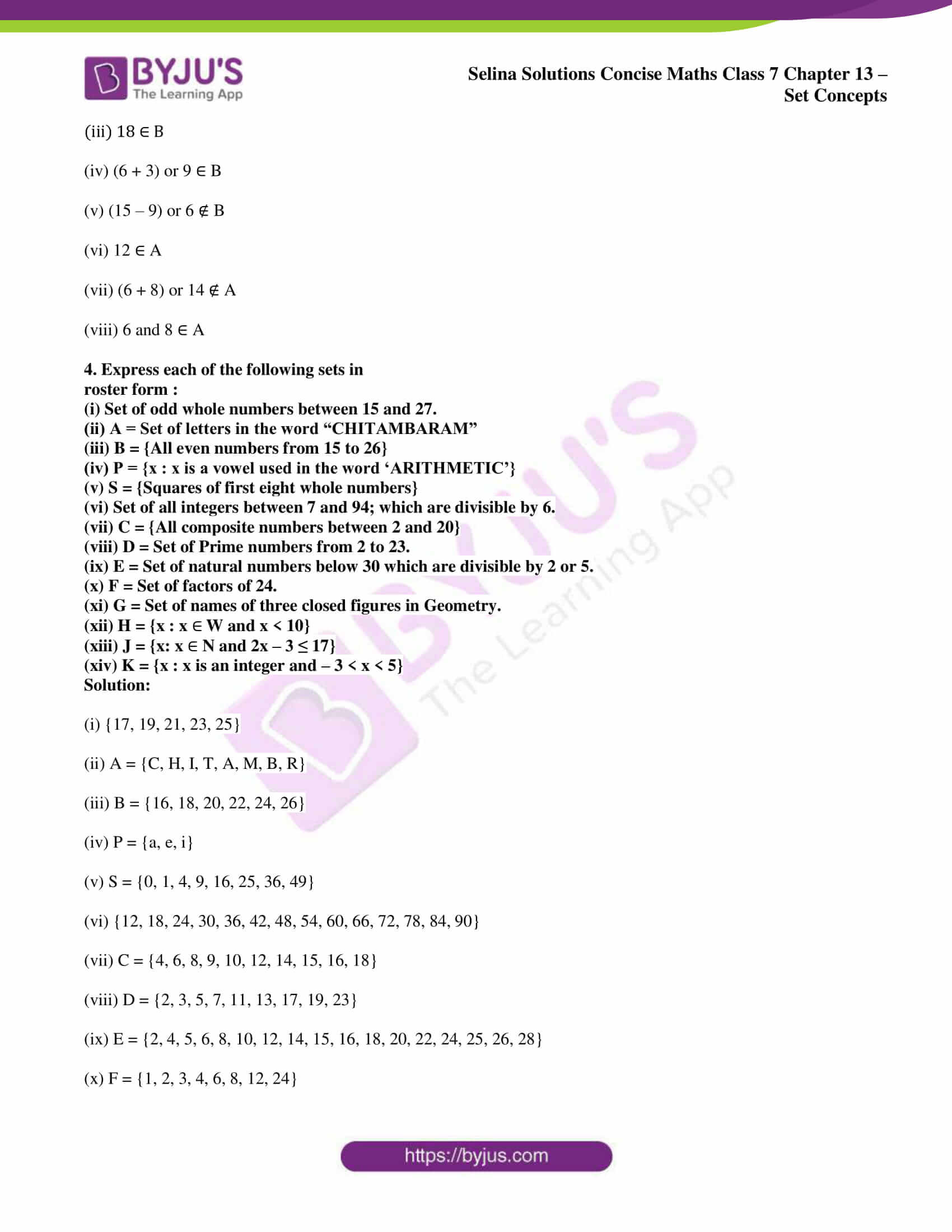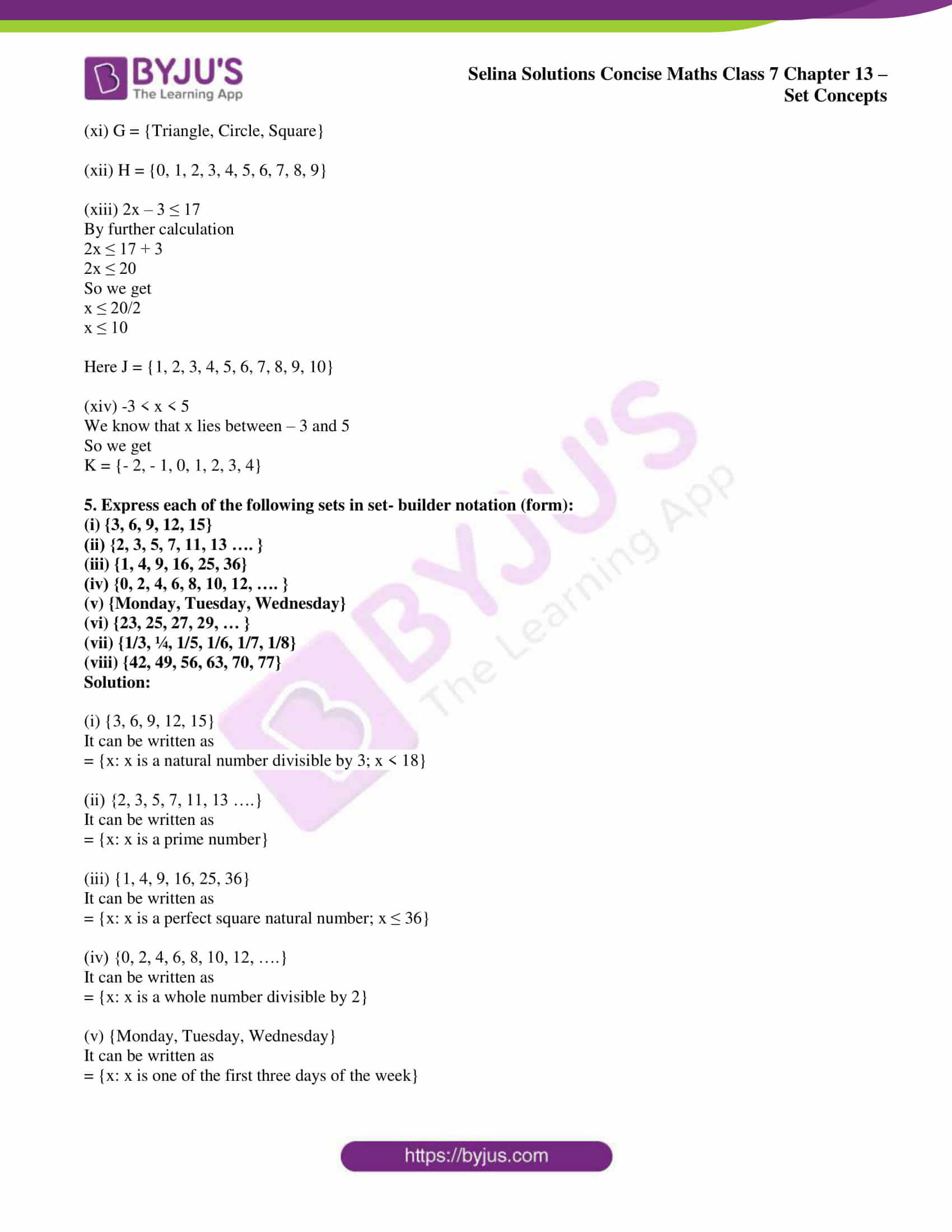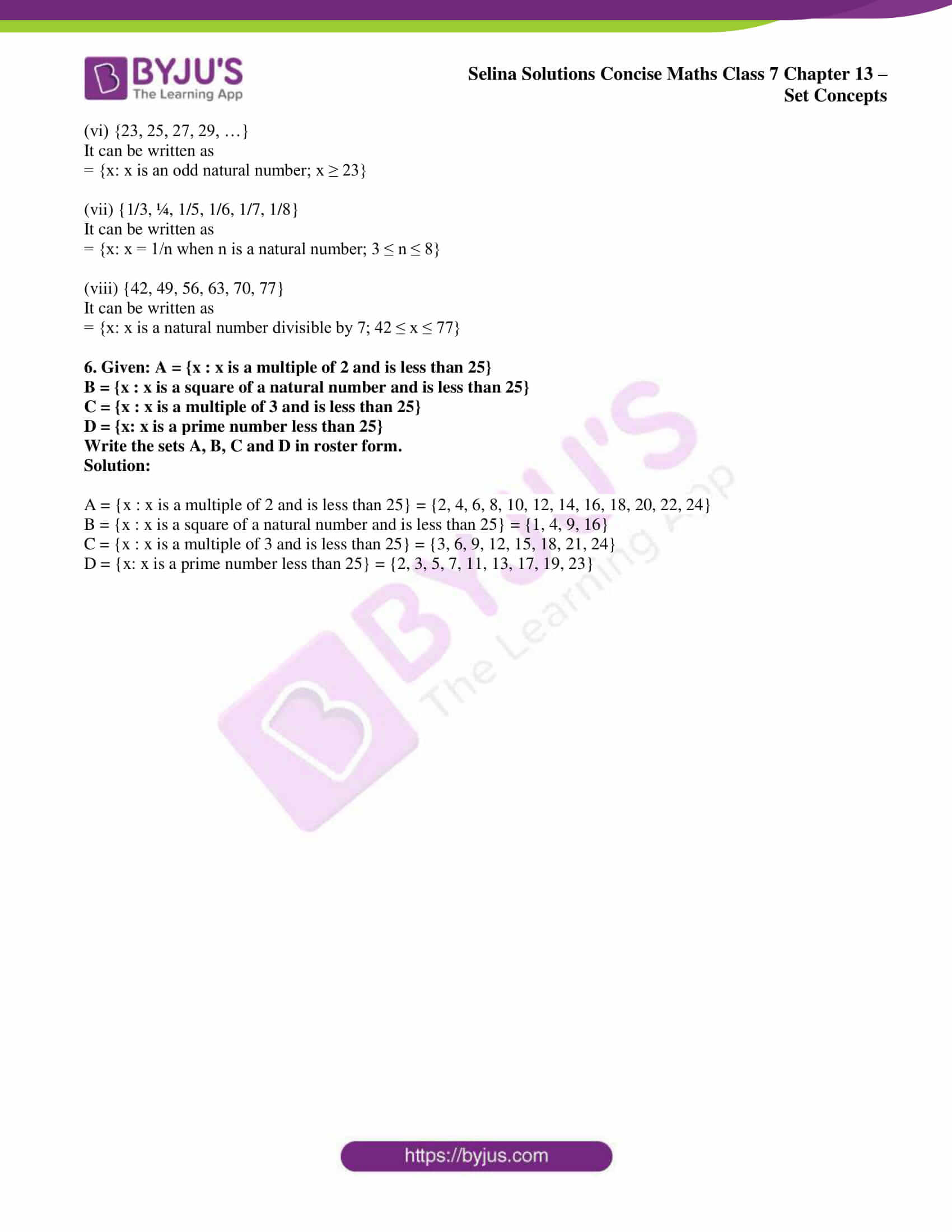# Selina Solutions Concise Maths Class 7 Chapter 13: Set Concepts Exercise 13A

Selina Solutions Concise Maths Class 7 Chapter 13 Set Concepts Exercise 13A explains the basic concept and the method of representation of a set. The solutions are available in PDF format to provide students a chance to practise the problems, without any time limits. The experts create solutions purely based on the current ICSE syllabus for Class 7. Each step is clearly explained to boost confidence among students in solving problems effortlessly. Here, the students can use Selina Solutions Concise Maths Class 7 Chapter 13 Set Concepts Exercise 13A PDF, from the links which are given below.

## Selina Solutions Concise Maths Class 7 Chapter 13: Set Concepts Exercise 13A Download PDF### Access other exercises of Selina Solutions Concise Maths Class 7 Chapter 13: Set Concepts

Exercise 13B Solutions

Exercise 13C Solutions

Exercise 13D Solutions

### Access Selina Solutions Concise Maths Class 7 Chapter 13: Set Concepts Exercise 13A

#### Exercise 13A page: 148

1. Find, whether or not, each of the following collections represent a set:

(i) The collection of good students in your school.

(ii) The collection of the numbers between 30 and 45.

(iii) The collection of fat-people in your colony.

(iv) The collection of interesting books in your school library.

(v) The collection of books in the library and are of your interest.

Solution:

(i) The collection of good students in your school is not a set as it is not well defined.

(ii) The collection of the numbers between 30 and 45 is a set.

(iii) The collection of fat-people in your colony is not a set as it is not well defined.

(iv) The collection of interesting books in your school library is not a set as it is not well defined.

(v) The collection of books in the library and are of your interest is a set.

2. State whether true or false:

(i) Set {4, 5, 8} is same as the set {5, 4, 8} and the set {8, 4, 5}
(ii) Sets {a, b, m, n} and {a, a, m, b, n, n) are same.

(iii) Set of letters in the word ‘suchismita’ is {s, u, c, h, i, m, t, a}
(iv) Set of letters in the word ‘MAHMOOD’ is {M, A, H, O, D}.

Solution:

(i) It is true.

(ii) It is true.

(iii) It is true as {s, u, c, h, i, s, m, i, t, a} = {s, u, c, h, i, m, t, a}

(iv) It is true as it has the same elements.

3. Let set A = {6, 8, 10, 12} and set B = {3, 9, 15, 18}.
Insert the symbol ‘∈ ’ or ‘ ∉ ’ to make each of the following true :
(i) 6 …. A
(ii) 10 …. B
(iii) 18 …. B
(iv) (6 + 3) …. B
(v) (15 – 9) …. B
(vi) 12 …. A
(vii) (6 + 8) …. A
(viii) 6 and 8 …. A

Solution:

(i) 6 ∈ A

(ii) 10 ∉ B

(iii) 18 ∈ B

(iv) (6 + 3) or 9 ∈ B

(v) (15 – 9) or 6 ∉ B

(vi) 12 ∈ A

(vii) (6 + 8) or 14 ∉ A

(viii) 6 and 8 ∈ A

4. Express each of the following sets in
roster form :
(i) Set of odd whole numbers between 15 and 27.
(ii) A = Set of letters in the word “CHITAMBARAM”
(iii) B = {All even numbers from 15 to 26}
(iv) P = {x : x is a vowel used in the word ‘ARITHMETIC’}
(v) S = {Squares of first eight whole numbers}
(vi) Set of all integers between 7 and 94; which are divisible by 6.
(vii) C = {All composite numbers between 2 and 20}
(viii) D = Set of Prime numbers from 2 to 23.
(ix) E = Set of natural numbers below 30 which are divisible by 2 or 5.
(x) F = Set of factors of 24.
(xi) G = Set of names of three closed figures in Geometry.
(xii) H = {x : x
W and x < 10}
(xiii) J = {x: x
N and 2x – 3 ≤ 17}
(xiv) K = {x : x is an integer and – 3 < x < 5}

Solution:

(i) {17, 19, 21, 23, 25}

(ii) A = {C, H, I, T, A, M, B, R}

(iii) B = {16, 18, 20, 22, 24, 26}

(iv) P = {a, e, i}

(v) S = {0, 1, 4, 9, 16, 25, 36, 49}

(vi) {12, 18, 24, 30, 36, 42, 48, 54, 60, 66, 72, 78, 84, 90}

(vii) C = {4, 6, 8, 9, 10, 12, 14, 15, 16, 18}

(viii) D = {2, 3, 5, 7, 11, 13, 17, 19, 23}

(ix) E = {2, 4, 5, 6, 8, 10, 12, 14, 15, 16, 18, 20, 22, 24, 25, 26, 28}

(x) F = {1, 2, 3, 4, 6, 8, 12, 24}

(xi) G = {Triangle, Circle, Square}

(xii) H = {0, 1, 2, 3, 4, 5, 6, 7, 8, 9}

(xiii) 2x – 3 ≤ 17

By further calculation

2x ≤ 17 + 3

2x ≤ 20

So we get

x ≤ 20/2

x ≤ 10

Here J = {1, 2, 3, 4, 5, 6, 7, 8, 9, 10}

(xiv) -3 < x < 5

We know that x lies between – 3 and 5

So we get

K = {- 2, – 1, 0, 1, 2, 3, 4}

5. Express each of the following sets in set- builder notation (form):
(i) {3, 6, 9, 12, 15}
(ii) {2, 3, 5, 7, 11, 13 …. }
(iii) {1, 4, 9, 16, 25, 36}
(iv) {0, 2, 4, 6, 8, 10, 12, …. }
(v) {Monday, Tuesday, Wednesday}
(vi) {23, 25, 27, 29, … }

(vii) {1/3, ¼, 1/5, 1/6, 1/7, 1/8}

(viii) {42, 49, 56, 63, 70, 77}

Solution:

(i) {3, 6, 9, 12, 15}

It can be written as

= {x: x is a natural number divisible by 3; x < 18}

(ii) {2, 3, 5, 7, 11, 13 ….}

It can be written as

= {x: x is a prime number}

(iii) {1, 4, 9, 16, 25, 36}

It can be written as

= {x: x is a perfect square natural number; x ≤ 36}

(iv) {0, 2, 4, 6, 8, 10, 12, ….}

It can be written as

= {x: x is a whole number divisible by 2}

(v) {Monday, Tuesday, Wednesday}

It can be written as

= {x: x is one of the first three days of the week}

(vi) {23, 25, 27, 29, …}

It can be written as

= {x: x is an odd natural number; x ≥ 23}

(vii) {1/3, ¼, 1/5, 1/6, 1/7, 1/8}

It can be written as

= {x: x = 1/n when n is a natural number; 3 ≤ n ≤ 8}

(viii) {42, 49, 56, 63, 70, 77}

It can be written as

= {x: x is a natural number divisible by 7; 42 ≤ x ≤ 77}

6. Given: A = {x : x is a multiple of 2 and is less than 25}
B = {x : x is a square of a natural number and is less than 25}
C = {x : x is a multiple of 3 and is less than 25}
D = {x: x is a prime number less than 25}
Write the sets A, B, C and D in roster form.

Solution:

A = {x : x is a multiple of 2 and is less than 25} = {2, 4, 6, 8, 10, 12, 14, 16, 18, 20, 22, 24}

B = {x : x is a square of a natural number and is less than 25} = {1, 4, 9, 16}

C = {x : x is a multiple of 3 and is less than 25} = {3, 6, 9, 12, 15, 18, 21, 24}

D = {x: x is a prime number less than 25} = {2, 3, 5, 7, 11, 13, 17, 19, 23}